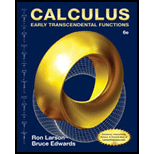Chapter 14.3, Problem 62ECalculus: Early Transcendental Fun...

6th Edition
Ron Larson + 1 other
ISBN: 9781285774770

Solutions

Chapter
SectionCalculus: Early Transcendental Fun...

6th Edition
Ron Larson + 1 other
ISBN: 9781285774770
Textbook Problem

Approximation:In Exercises 59 and 60, use a computer algebra system to approximate the iterated integral. ∫ 0 π 4 ∫ 0 4 5 r e r θ d r d θ

To determine

To calculate: The approximate value of the double integral 0π4045rerθdrdθ using computer algebra system.

Explanation

Given: 0π4045re<

Still sussing out bartleby?

Check out a sample textbook solution.

See a sample solution

The Solution to Your Study Problems

Bartleby provides explanations to thousands of textbook problems written by our experts, many with advanced degrees!

Get Started

Find more solutions based on key concepts